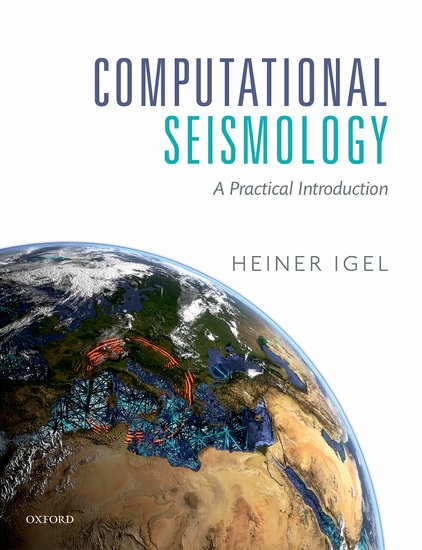Computational Seismology
The Fourier Pseudospectral Method - Acoustic Waves in 1DThis notebook is part of the supplementary material to Computational Seismology: A Practical Introduction, Oxford University Press, 2016.

## Basic Equations¶

We use the Fourier method to calculate exact n-th derivatives on a regular spaced grid (to machine precision). This property combined with classical time extrapolation schemes result in the so call Fourier pseudospectral method. The problem of solving the 1D acoustic wave equation in an homogeneous media

\begin{equation} \partial_t^2 p(x,t) = c(x)^2 \ \partial_x^2 p(x,t) + s(x,t) \end{equation}

is covered in this notebook. We explore the benefits of calculating exact spatial derivatives (up to machine precision), numerical dispersion, comparison with a Finite Difference scheme

In :
# This is a configuration step for the exercise. Please run it before calculating the derivative!
import numpy as np
import matplotlib
# Show Plot in The Notebook
matplotlib.use("nbagg")
import matplotlib.pyplot as plt
from ricker import ricker


### 1. Fourier derivative method¶

The second spatial derivative is computed by multiplying the spatial Fourier transform of the pressure field $P(k,t)$ with $(ik)^2$

\begin{equation} \partial_x^2 p(x,t) = \mathscr{F}^{-1}[(ik)^{2}P(k,t)] = \frac{1}{\sqrt{2\pi}} \int_{-\infty}^{\infty} (ik)^{2} P(k,t) e^{ikx} dk \end{equation}

where $k$ is the wavenumber and $IFT$ the Inverse Fourier Transform. A function to perform this task is implemented in the next cell.

In :
def fourier_derivative_2nd(f, dx):
# Length of vector f
nx = np.size(f)
# Initialize k vector up to Nyquist wavenumber
kmax = np.pi / dx
dk = kmax / (nx / 2)
k = np.arange(float(nx))
k[: int(nx/2)] = k[: int(nx/2)] * dk
k[int(nx/2) :] = k[: int(nx/2)] - kmax

# Fourier derivative
ff = np.fft.fft(f)
ff = (1j*k)**2 * ff
df_num = np.real(np.fft.ifft(ff))
return df_num


### 2. Initialization of setup¶

In :
# Basic parameters
# ---------------------------------------------------------------
nt    = 3500    # number of time steps
c     = 343.    # acoustic velocity [m/s]
eps   = 0.2     # stability limit
isnap = 50      # snapshot frequency
isx   = 1250    # source location
f0    = 60.     # Frequency [Hz](div by 5)
nx    = 2024    # number of grid points in x

# pressure fields Initialization
p = np.zeros(nx) ; pnew = p ; pold = p ; d2p = p; dp = p
ap = np.zeros(nx); apnew = ap; apold = ap; ad2p = ap; adp = ap
sp = np.zeros(nx); spnew = sp; spold = sp; sd2p = sp; sdp = p

dx = 1250./(nx-1)  # calculate space increment
x  = np.arange(0, nx)*dx   # initialize space coordinates
dt = eps*dx/c; # calculate time step from stability criterion


### 3. Source Initialization¶

In :
# source time function
# ---------------------------------------------------------------
t   = np.arange(1, nt+1)*dt  # initialize time axis
T0  = 1./f0
tmp = ricker(dt, T0)
tmp = np.diff(tmp)
src = np.zeros(nt)
src[0:np.size(tmp)] = tmp
lam = c*T0

#spatial source function
# ---------------------------------------------------------------
sigma = 2*dx
x0 = x[isx-1]
sg = np.exp(-1/sigma**2 *(x - x0)**2); sg = sg/np.amax(sg)


### 4. Time Extrapolation¶

The final solution for our 1D acoustic wave problem after introducing a finite differences time extrapolation schem can be written as

\begin{equation} p_{j}^{n+1} = dt^2c_{j}^{2} \partial_{x}^{2}p_{j}^{n} + dt^2s_{j}^{n} + 2p_{j}^{n} - p_{j}^{n-1} \end{equation}

where the space derivative is computed with the Fourier method. In order to compare the above numerical solution, we implement a 3-point finite difference operator, as well as a 5-point finite difference operator to compute spatial derivatives. They are given as:

1.) 3-point finite difference operator \begin{equation} \partial_x^2 p(x,t) = \frac{p(x+\mathrm{d}x,t) - 2 p(x,t) + p(x-\mathrm{d}x,t)}{\mathrm{d}x^2} \end{equation}

2.) 5-point finite difference operator \begin{equation} \partial_x^2 p(x,t) = \frac{-p(x+2\mathrm{d}x,t) + 16p(x+\mathrm{d}x,t) - 30p(x,t) + 16p(x-\mathrm{d}x,t) - p(x-2\mathrm{d}x,t)}{12\mathrm{d}x^2} \end{equation}

#### Numerical dispersion¶

One of the most prominent characteristic of the Fourier method is the low numerical dispersion in comparison with the finite difference method. The animation displayed below compare the effects of numerical dispersion on the solution of the 1D acoustic equation using our three different approaches.

In :
# Initialize animated plot
# ---------------------------------------------------------------
fig, (ax1, ax2, ax3) = plt.subplots(3, sharex=True, sharey=True, figsize=(12,7))
line1 = ax1.plot(x[isx:], p[isx:], 'k', lw=1.5, label='FD-3pt')
line2 = ax2.plot(x[isx:], ap[isx:], 'r', lw=1.5, label='FD-5pt')
line3 = ax3.plot(x[isx:], sp[isx:], 'b', lw=1.5, label='Fourier')
ax1.axis([isx*dx, nx*dx, -6E-7, 6E-7]); ax3.set_xlabel('x [m]')
ax1.legend(loc=4)
ax2.legend(loc=4)
ax3.legend(loc=4)

plt.ion()   # set interective mode
plt.show()

# ---------------------------------------------------------------
# Time extrapolation
# ---------------------------------------------------------------
for it in range(nt):
# ----------------------------------------
# Fourier Pseudospectral Method
# ----------------------------------------
sd2p  = fourier_derivative_2nd(sp, dx)      # 2nd space derivative
spnew = 2*sp - spold + c**2 * dt**2 * sd2p  # Time Extrapolation
spnew = spnew + sg*src[it]*dt**2            # Add sources
spold, sp = sp, spnew                       # Time levels
sp = 0; sp[nx-1] = 0     # set boundaries pressure free

# ----------------------------------------
# Finite Differences Method 3pt
# ----------------------------------------
for i in range(1, nx-1):
d2p[i] = (p[i+1] - 2*p[i] + p[i-1])/dx**2 # Space derivative
pnew = 2*p - pold + dt**2 * c**2 * d2p # Time Extrapolation
pnew = pnew + sg*src[it]*dt**2 # Add source
pold, p = p, pnew # Time levels
p = 0; p[nx-1] = 0 # set boundaries pressure free

# ----------------------------------------
# Finite Differences Method 5pt
# ----------------------------------------
for i in range(2, nx-2):
ad2p[i] = (-1/12*ap[i+2] + 4/3*ap[i+1] - 5/2*ap[i] \
+ 4/3*ap[i-1] - 1/12*ap[i-2])/dx**2 # Space derivative
apnew = 2*ap - apold + dt**2 * c**2 * ad2p # Time Extrapolation
apnew = apnew + sg*src[it]*dt**2 # Add source
apold, ap = ap, apnew # Time levels
ap = 0; ap[nx-1] = 0 # set boundaries pressure free

# --------------------------------------
# Animation plot. Display solution
if not it % isnap:
for l in line1:
l.remove()
del l
for l in line2:
l.remove()
del l
for l in line3:
l.remove()
del l

# --------------------------------------
# Display lines
line1 = ax1.plot(x[isx:], p[isx:], 'k', lw=1.5)
line2 = ax2.plot(x[isx:], ap[isx:], 'r', lw=1.5)
line3 = ax3.plot(x[isx:], sp[isx:], 'b', lw=1.5)
plt.gcf().canvas.draw()